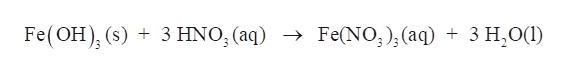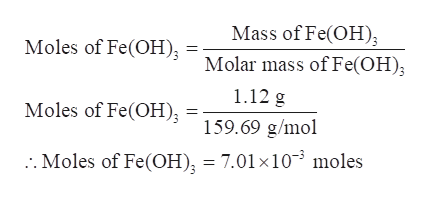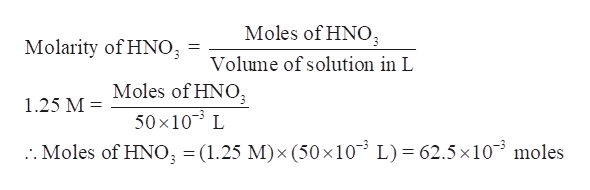50.0 mL of a 1.25 M solution of nitric acid is added to 1.12 g of solid iron(III) hydroxide, what is the concentration (in M) of iron(III) ions in the final solution?Fe(OH)3(s)  +  3 HNO3(aq)  →  Fe(NO3)3(aq)  +  3 H2O(l)Assume the volume of the solution remains constant. Do not include the units in your answer.  Your answer should have three significant figures.

Question

50.0 mL of a 1.25 M solution of nitric acid is added to 1.12 g of solid iron(III) hydroxide, what is the concentration (in M) of iron(III) ions in the final solution?

Fe(OH)3(s)  +  3 HNO3(aq)  →  Fe(NO3)3(aq)  +  3 H2O(l)

Assume the volume of the solution remains constant. Do not include the units in your answer.  Your answer should have three significant figures.

Step 1

50.0 mL of a 1.25 M solution of nitric acid is added to 1.12 g of solid iron(III) hydroxide, the concentration (in M) of iron(III) ions in the final solution is to be calculated assuming the volume of the solution remains constant.help_outlineImage TranscriptioncloseFe(OH), (s) 3 HNO, (aq) > Fe(NO) (aq) + 3 H,O() fullscreen
Step 2

Calculate the moles of Iron (III) oxide, Fe(OH)3, in 1.12 g of solid iron(III) hydroxide -

Given: Mass of Fe(OH)3 = 1.12 g

Molar mass of Fe(OH)3 = 159.69 g/molhelp_outlineImage TranscriptioncloseMass of Fe(OH) Moles of Fe(OFH); Molar mass of Fe(OH) 1.12 g Moles of Fe(OH); 159.69 g/mol . Moles of Fe(OH) = 7.01 x10 moles fullscreen
Step 3

Calculate the moles of nitric acid-

Molarity of HNO3 = 1.25 M

Volume ...help_outlineImage TranscriptioncloseMoles of HNO Molarity of HNO Volume of solution in L Moles of HNO 50x10 L :. Moles of HNO3 = (1.25 M)x (50 x10 1.25 M L) = 62.5x10 moles fullscreen

Want to see the full answer?

See Solution

Want to see this answer and more?

Our solutions are written by experts, many with advanced degrees, and available 24/7

See Solution
Tagged in

Chemistry